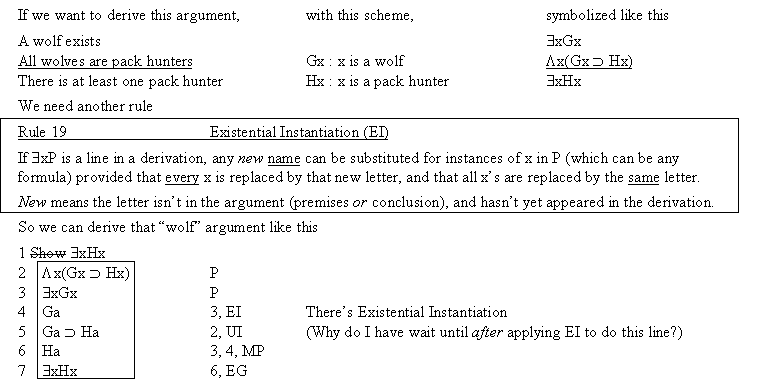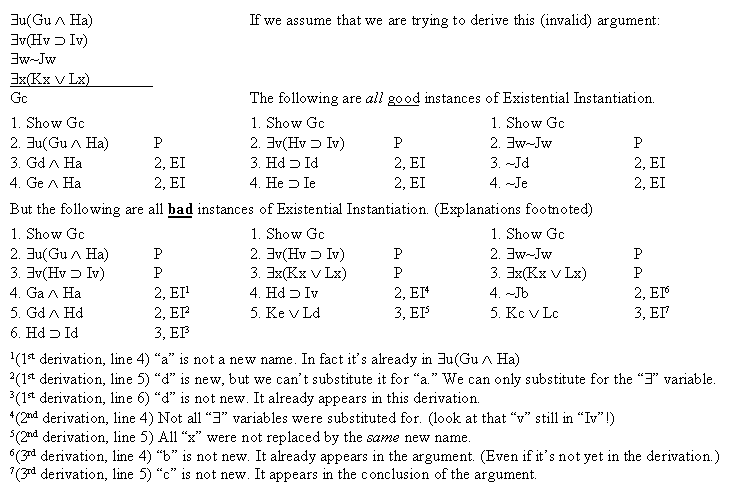Logic Chapter Twenty ThreeQuiz preparation: Mark the following true or false based on the definition of UG above.
1. In EI, any name can be substituted for instances of x in P.
2. In EI, any name that isn't already in the derivation can be substituted for instances of x in P.
3. In EI, any name that isn't in the argument's premises can be substituted for instances of x in P.
4. In EI, any name that isn't in the argument's conclusion can be substituted for instances of x in P.
5. In EI, any name that isn't in the argument can be substituted for instances of x in P.
6. In EI, any name that isn't in the argument or the derivation so far can be substituted for instances of x in P.
7. In EI, any variable can be replaced.
8. In EI, only the existential variable can be replaced.
9. In EI, any instance of the existential variable can be replaced.
10. In EI, all instances of the existential variable must be replaced.
11. In EI, each instance of the existential variable can be replaced with a different name.
12. In EI, each instance of the existential variable must be replaced with the same name.